Courses

# Test: Cross Product

## 5 Questions MCQ Test Mathematics (Maths) Class 12 | Test: Cross Product

Description
This mock test of Test: Cross Product for JEE helps you for every JEE entrance exam. This contains 5 Multiple Choice Questions for JEE Test: Cross Product (mcq) to study with solutions a complete question bank. The solved questions answers in this Test: Cross Product quiz give you a good mix of easy questions and tough questions. JEE students definitely take this Test: Cross Product exercise for a better result in the exam. You can find other Test: Cross Product extra questions, long questions & short questions for JEE on EduRev as well by searching above.
QUESTION: 1

### If | a | = 2, | b | = 5 and | a × b | = 8, then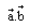can be equal to

Solution: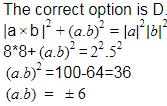QUESTION: 2

### If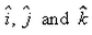are unit vectors along X-axis, Y-axis and Z-axis respectively, then

Solution:

As we know that,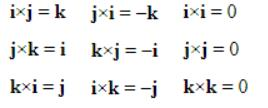QUESTION: 3

### The area of triangle whose adjacent sides are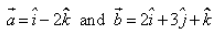is :

Solution:

Area of triangle = ½(a * b)
a = (1, 0, -2)   b = (2, 3, 1)
= i(0 + 6) + j(-4 - 1) + k(3 - 0)
= 6i - 5j + 3k
|a * b| = (36 + 25 + 9)½
|a * b| = (70)½
Area of triangle = ½(a * b)
= [(70)½]/2

QUESTION: 4

The value of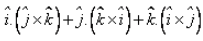is:

Solution:

i.(j * k) + j.(k * i) + k(i * j)
= i.(i) + j.(j) + k.(k)
= 1 + 1 + 1
= 3

QUESTION: 5

The area of parallelogram whose sides are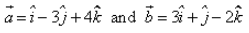is:​

Solution:

A = {(1, -3, 4)}    B = {(3, 1, -2)}
A * B = {(i^(6-4) - j^(-2 -12) + k^ (1+9)}
= 2i^ + 14j^ + 10k^
Area = [(2)2 + (14)2 + (10)2]½
= (300)½
= 10(3)½ sq unit